# In the circuit shown, the current through theresistor isa)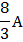b)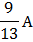c)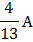d)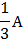## Question ID - 150549 :- In the circuit shown, the current through theresistor isa)b)c)d)3537

(d)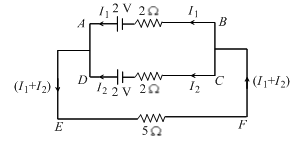Applying Kirchhoff’s second law for closed loopWe getor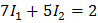…(i)

Again, applying Kirchhoff’s second law for a closed loop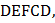we getor…(ii)

Multiplying (i) by 5 and (ii) by 7, we get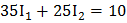…(iii)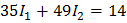…(iv)

Subtracting (iv) from (iii) we get,Substituting the value of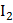in equation (i), we get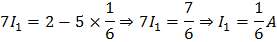The current through theisNext Question :
 A 5.0 A current is setup in an external circuit by a 6.0 storage battery for 6.0 min.  The chemical energy of the battery is reduced by a)b)c)d)